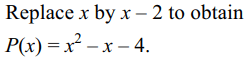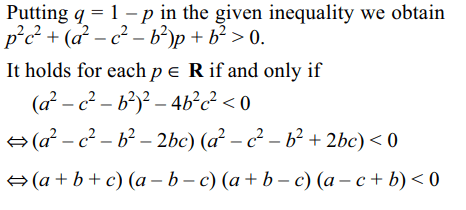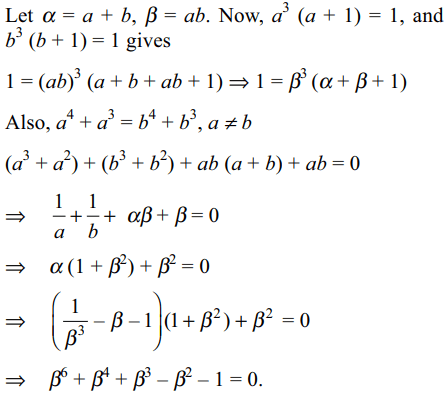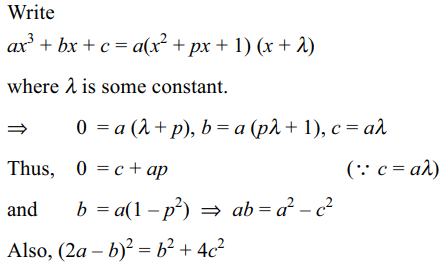1. Let P(x) be a polynomial such that $P \left(x+2 \right)=x^{2}+3x-2$     . Which one of the followings are true statement?
a) Product of the roots of P(x) = 0 is – 4
b) P(x) must be of degree 2
c) P(x) = 0 has two irrational roots
d) All of the above

Explanation:2. Let P(x) be a polynomial such that $P \left(x^{2}+2 \right)=x^{17}-3x^{5}+x^{3}-3$      , then
a) P(x) = 0 has at least 34 roots
b) P(x) = 0 has exactly 17 roots
c) Sum of the roots of P(x) = 0 is – 193
d) none of these

Explanation:3. Let $P \left(x \right)=a_{0}+a_{1}x+...+a_{n}x^{n},a_{n}\neq 0$        be such that $P \left(x^{2} \right)=P (x)^{2}$   , then
a) $a_{0}=1$
b) $a_{0}=a_{1}=....=a_{n-1}=0$
c) $a_{n}=1$
d) Both b and c

Explanation:4. Let p, q be non-zero, real numbers and $x_{1},x_{2},x_{3}$  be the roots of $x^{3}+px+q=0$    such that $x_{3}=\frac{1}{x_{1}}+\frac{1}{x_{2}}$    then
a) q is a root of $x^{2}+px+q=0$
b) q < 0
c) p < 0
d) Both a and c

Explanation:5. Let $n\epsilon N, n>1$  and $\alpha\epsilon R$  be such that sin$\alpha \neq 0$   , and let $p\left(x\right)=x^{n}\sin\alpha -x \sin n\alpha + \sin \left(n-1\right) \alpha$
then
a) $\cos\alpha \pm 1\sin \alpha$    are zeros of p(x)
b) p(x) is divisible by $x^{2}-2x \cos\alpha+1$
c) p(x) is divisible by $x^{2}+2x \cos\alpha+1$
d) Both a and b

Explanation:6. Let $n \epsilon N, n>1$   and $\alpha \epsilon R$  be such that $\sin\alpha\neq 0$     and let$p\left(x\right)=\left(x\sin\alpha+\cos\alpha\right)^{n}-x\sin\left(n\alpha\right)-\cos\left(n\alpha\right)$
then
a) $\pm$ i are zeros of p(x)
b) p(x) is divisible by $x^{2}+1$
c) $\pm$ 1 are zeros of p(x)
d) Both a and b

Explanation: p(x) is divisible by $x^{2}+1$

7. Let a, b, c > 0 and for all $p,q \epsilon R$  with p + q = 1
$pa^{2}+qb^{2}>pbc^{2}$     , then
a) a + b > c
b) a + c > b
c) b + c > a
d) All of the above

Explanation:8. Let a, b be two distinct roots of $x^{4}+x^{3}-1=0$     , and p(x) = $x^{6}+x^{4}+x^{3}-x^{2}-1$
a) ab is a root of p(x) = 0
b) a + b is a root of p(x) = 0
c) both a + b and ab are roots of p(x) = 0
d) none of ab, a + b is a root of p(x) = 0

Explanation:9. If $x^{2}+px+1$   is a factor of $ax^{3}+bx+c$    , then
a) ax + c is also a factor of $ax^{3}+bx+c$
b) ap+c=0
c) $a^{2}-c^{2}=ab$
d) All of the above10. Let a, b, c, d and p be integers such that $p\neq 0$   . If x + p is a factor of $ax^{3}+bx^{2}+cx+d$     , then
a) $p\mid d$
b) $p^{2}\mid \left(pc-d \right)$
c) $d=0\Rightarrow p$   is a root of $ax^{2}-bx+c=0$